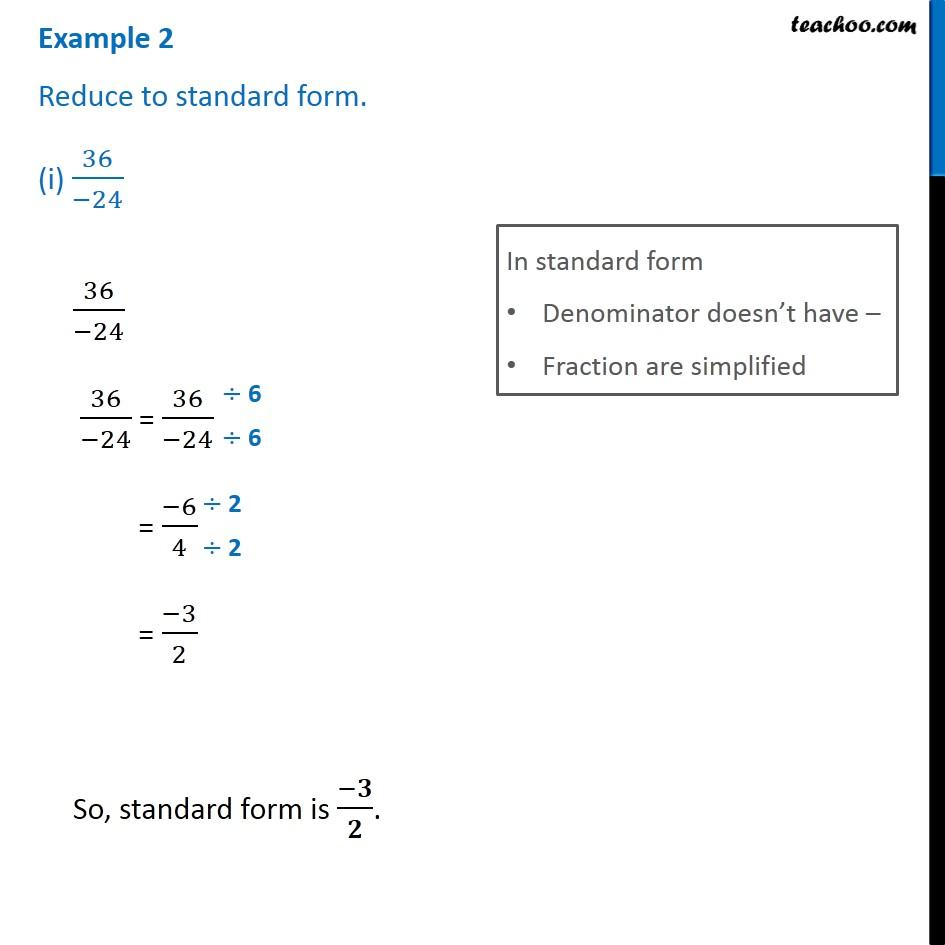Standard form of rational numbers

Chapter 9 Class 7 Rational Numbers
Concept wiseIntroducing your new favourite teacher - Teachoo Black, at only ₹83 per month

### Transcript

Reduce to standard form. (i) 36/(−24) In standard form Denominator doesn’t have – Fraction are simplified 36/(−24) 36/(−24) = 36/(−24) = (−6)/4 = (−3)/2 So, standard form is (−𝟑)/𝟐.